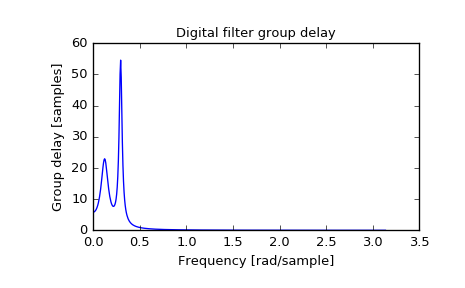# scipy.signal.group_delay¶

scipy.signal.group_delay(system, w=None, whole=False)[source]

Compute the group delay of a digital filter.

The group delay measures by how many samples amplitude envelopes of various spectral components of a signal are delayed by a filter. It is formally defined as the derivative of continuous (unwrapped) phase:

          d        jw
D(w) = - -- arg H(e)
dw

Parameters: system : tuple of array_like (b, a) Numerator and denominator coefficients of a filter transfer function. w : {None, int, array-like}, optional If None (default), then compute at 512 frequencies equally spaced around the unit circle. If a single integer, then compute at that many frequencies. If array, compute the delay at the frequencies given (in radians/sample). whole : bool, optional Normally, frequencies are computed from 0 to the Nyquist frequency, pi radians/sample (upper-half of unit-circle). If whole is True, compute frequencies from 0 to 2*pi radians/sample. w : ndarray The normalized frequencies at which the group delay was computed, in radians/sample. gd : ndarray The group delay.

freqz
Frequency response of a digital filter

Notes

The similar function in MATLAB is called grpdelay.

If the transfer function $$H(z)$$ has zeros or poles on the unit circle, the group delay at corresponding frequencies is undefined. When such a case arises the warning is raised and the group delay is set to 0 at those frequencies.

For the details of numerical computation of the group delay refer to [R203].

References

 [R203] (1, 2) Richard G. Lyons, “Understanding Digital Signal Processing, 3rd edition”, p. 830.

Examples

>>> from scipy import signal
>>> b, a = signal.iirdesign(0.1, 0.3, 5, 50, ftype='cheby1')
>>> w, gd = signal.group_delay((b, a))

>>> import matplotlib.pyplot as plt
>>> plt.title('Digital filter group delay')
>>> plt.plot(w, gd)
>>> plt.ylabel('Group delay [samples]')